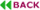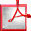doi: 10.7873/DATE.2015.0158

# Formal Verification of Sequential Galois Field Arithmetic Circuits Using Algebraic Geometry

#### 1Electrical & Computer Engineering, University of Utah, Salt Lake City, USA.

axiaojuns@ece.utah.edu
bkalla@ece.utah.edu
ctpruss@ece.utah.edu

#### 2Mathematics & Statistics, Georgia State University, Atlanta, USA.

fenescu@mathstat.gsu.edu

## ABSTRACT

Sequential Galois field (\mthbbF2k) arithmetic circuits take k-bit inputs and produce a k-bit result, after k-clock cycles of operation. Formal verification of sequential arithmetic circuits with large datapath size is beyond the capabilities of contemporary verification techniques. To address this problem, this paper describes a verification method based on algebraic geometry that: i) implicitly unrolls the sequential arithmetic circuit over multiple (k) clock-cycles; and ii) represents the function computed by the state-registers of the circuit, canonically, as a multi-variate word-level polynomial over \mthbbF2k. Our approach employs the Gröbner basis theory over a specific elimination ideal. Moreover, an efficient implementation is described to identify the k-cycle computation performed by the circuit at word-level. We can verify up to 100-bit sequential Galois field multipliers, whereas conventional techniques fail beyond 23-bit circuits.Full Text (PDF)